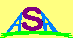### A SIMPLE D-REGION IONISATION MODEL

The model described here attempts to estimate the ionospheric D-region ionisation at around the 85 km altitude level. The algorithms describing this Basic Operational D-Region Ionisation (BODRI) model are presented below.

It is assumed that the electron density at 85 km is governed by 3 processes:

NDe = B + S + P
where B is the background component due to meteors , galactic cosmic rays and other sources. S is the solar component due to Lyman-alpha and X-rays. P is a component due to solar and radiation belt particle precipitation. This is significant mostly at high latitudes and has been ignored here.

The background component is given by:

B = 108 ( 1 + Rs / 100 )  [electrons/m3]
where Rs is the smoothed sunspot number.

The solar electromagnetic component is specified by:

S = [ m ( X + α L ) cos0.75( z ) ]n   [electrons/m3]
where X is the solar X-ray irradiance (0.1 to 0.8 nm) in Watts per square metre, α is the Lyman-alpha ionising efficiency (relative to the soft X-rays), z is the solar zenith angle, and m and n are model calibration factors.

L is the Lyman-alpha irradiance given by:

L = 0.0021 + 7.7 x 10-6 Rs + 3.8 x 10-6 R   [W/m2]
Initial model calibration sets α = 7 x 10-4, m = 2.7 x 1011 and n = 1.

The solar declination at any point on the Earth's surface is specified by:

cos ( z ) = sin ( μi ) sin ( δ ) + cos ( δ ) cos ( μi ) cos ( λs - λi )
where μi is the ionospheric latitude, λi is the ionospheric longitude, λs is the longitude of the sub-solar point and δ is the solar declination.

Solar longitude may be approximated to better than 2% by the formula:

λs = 15 ( 12 - UT )
where UT is the Universal Time in hours ( 0 to 24 ).
Solar declination may also be approximated (to better than 1%) by:
δ (degrees) = 23.5 sin ( YD - 82 )
where YD is the day of the year (1 to 365).
The model inputs are thus the ionospheric location (latitude and longitude), time and date, sunspot number (both instantaneous and smoothed), and instantaneous solar soft X-ray flux (0.1 - 0.8 nm) at the time and date in question.

The model output is the electron density (at 85 km altitude) in electrons per cubic metre, at the time and date and ionospheric location specified. The accuracy is unspecified at this time, and the model is obviously not applicable to polar regions.Australian Space Academy